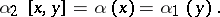# Baer multiplication

(diff) ← Older revision | Latest revision (diff) | Newer revision → (diff)

A binary operation on the set of classes of extensions of modules, proposed by R. Baer . Letandbe arbitrary modules. An extension ofwith kernelis an exact sequence: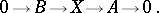(1)

The extension (1) is called equivalent to the extension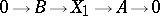if there exists a homomorphism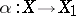forming part of the commutative diagram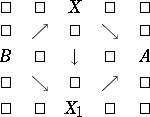The set of equivalence classes of extensions is denoted by. The Baer multiplication on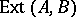is induced by the operation of products of extensions defined as follows. Let(2)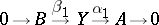(3)

be two extensions. In the direct sum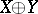the submodules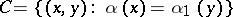andare selected. Clearly,, so that one can define the quotient module. The Baer product of the extensions (2) and (3) is the extension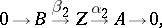whereand